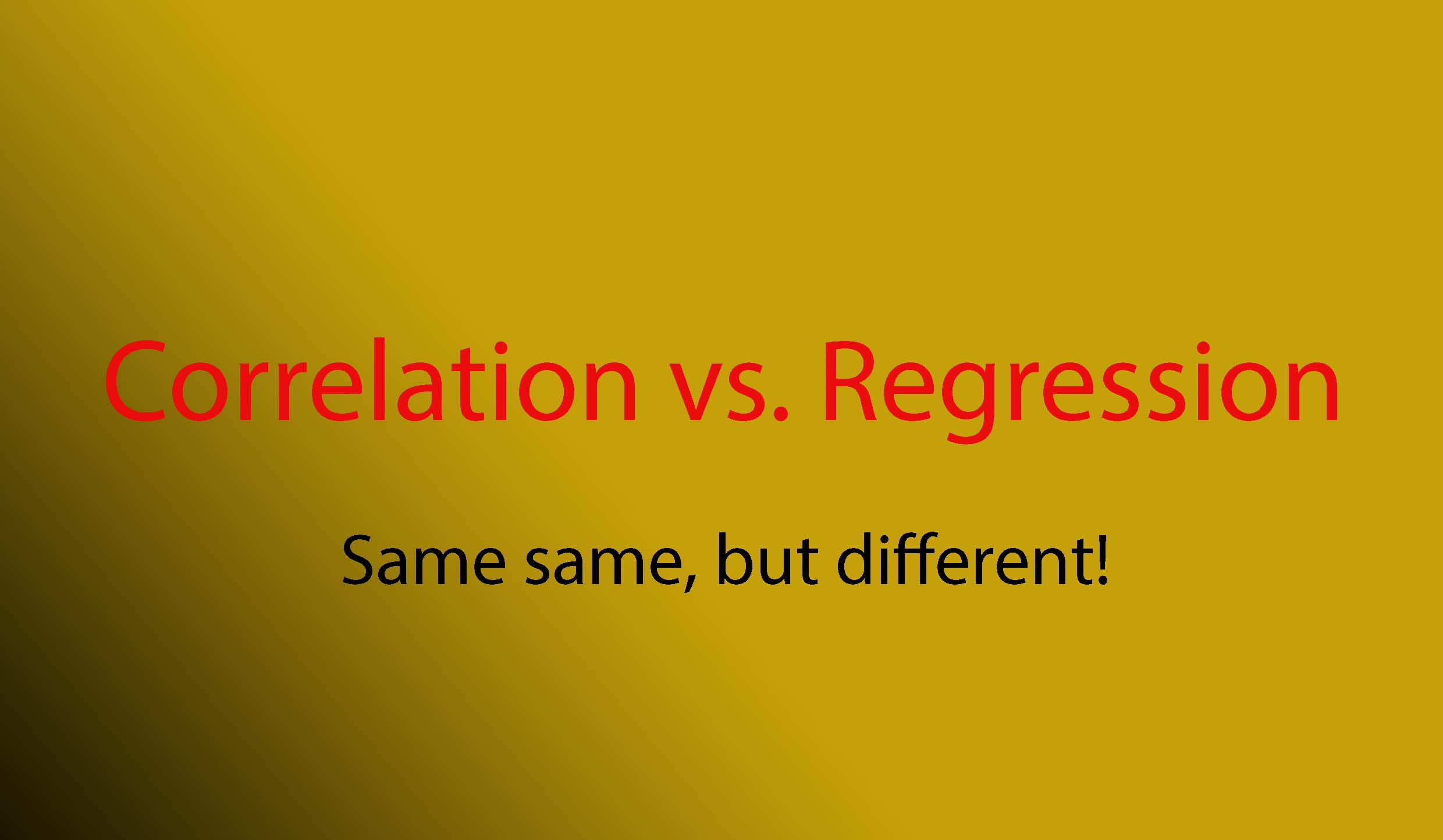# Correlation vs. Regression: Same-same, but different!Often, when we read a research article with a correlational design, the result was totally different, which is a prediction model. Mostly the researchers conducted two orders of analyses (a correlational analysis, followed by regression)

There is nothing wrong in terms of statistical perspectives but confusing in terms of the objectives, backgrounds, and principles.

Let me give you a perspective at a glance of the difference between the two designs rationally.

### Correlation

Correlation is primarily used to quickly and concisely summarize the direction, magnitude, and strength of the relationships between a set of 2 or more numeric variables.

Correlation quantifies the direction and strength of the relationship between two numeric variables, X and Y, and always lies between -1.0 and 1.0.

Rationales for a correlational study (You need to write in the background):

• No studies conducted a correlational study on the target topic
• There are existing studies, but inconsistent findings, such as:

(positive vs. negative);

(significant vs. non-significant correlation);

(small vs. big effect size);

• There are existing studies, but with small sample size or limited setting or different setting

### Regression

Regression is primarily used to build models/equations to predict a key response, Y, from a set of predictor (X) variables. We want to know how much power the independent variables affect the dependent variable, or it presents a causal effect.

Simple linear regression relates X to Y through an equation of the form Y = a + bX.

Rationales for a regression study (You need to write in the background)

• The significant correlations between independent and dependent variables should be clearly stated in the background.
• There are existing studies but in different settings. You believe the results will be different between hospital and public health center settings.
• There are existing studies but with a different model of regression. You believe with another regression model, the results will be a good fit.

Seeing the rationales between the two designs above, if you combine both, it will definitely create confusion. If we are talking about a research project, that would be fine because there are many objectives and designs. Combining both is common, sometimes more than two designs. But, if we are talking about a research article, the purpose, title, results, discussion, and conclusion should be in line. Therefore, no doubt, many reviewers often ask the researchers whether they would like to focus on correlation or regression.

Hopefully, it helps. Another perspective is welcomed.

Cite this as: Gunawan, J. (2021). Correlation vs. regression. Retrieved from: https://jokogunawan.com/blog/2021/07/19/research/735/